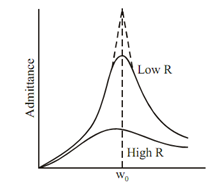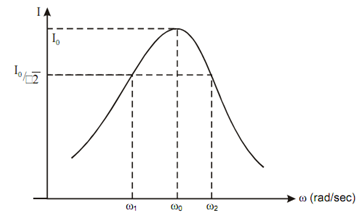#### All Pages

Assignment Help: >> Series and Parallel Resonance - Admittance Curve

Admittance Y is reciprocal of impedance. Its variation with frequency is illustrated in Figure

Y = 1 /ZFigure illustrates the variation of current with frequency. Current is maximum at resonance frequency ω. At rms value of current   I0 /√2 , power becomes half of its maximum value. Thus the points, corresponding to I/√2 on the resonance curve, are known as the half power points. Frequency ω1 and ω2 corresponding to these two points are known as the lower and upper half power frequency.Figure: Resonance Curve